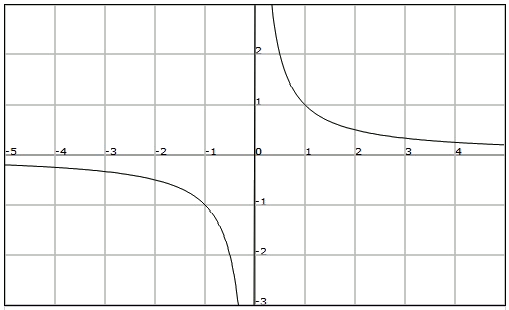# Rational functions

A rational function is a function whose analytic expression is given by a quotient between polynomials: $$\displaystyle f(x)=\frac{P(x)}{Q(x)}$$$In this type of function it is possible to calculate the image of any real number except those that are solutions of the denominator, since we will have to divide by $$0$$ and thus we do not obtain a real number. Therefore we can define the domain of this type of functions by $$Dom(f)=\mathbb{R}-\{x \in \mathbb{R} \mid Q(x)=0 \}$$$ An important case of rational function is the function of inverse proportionality: $$\displaystyle f(x)=\frac{k}{x}$$ where $$k$$ is a constant. Note that it is a rational function with $$P(x)=k\neq0$$ and $$Q(x)=x$$.

Its domain is the set of the real numbers that are not solutions to the denominator, that is, $$Dom (f) =\mathbb{R} - \{0\}$$

Its image is the set of real numbers except zero, since it is not an image of any element of the domain; that is, $$Im (f) =\mathbb{R}- \{0\}$$

Let's see the graph of the function $$\displaystyle f(x)=\frac{1}{x}$$: